## Approximated Spatial Masks and ReLu¶

Because the DCT is a linear transformation, often only other linear transformations of the image pixels can be reformulated to work on DCT coefficients. Smith and Rowe extended these linear transformations to include moving and choosing pixels based on a simple linear rule, but there is still no known methods for non-linear functions.

Here we introduce the Approximated Spatial Mask algorithm. This algorithm allows piecewise linear transformations to be applied to DCT coefficients. The algorithm is approximate and can be tuned to run slower for a more accurate result. The ASM is applied using a half-spatial mask application algorithm, which is able to apply a spatial domain mask directly to DCT coefficients.

The algorithm is demonstrated by formulating the ReLu function.

As usual what follows is boilerplate and can be ignored.

In :
!pip install tabulate

import numpy as np
import scipy.fftpack
import scipy.signal
from IPython.display import display, HTML
from tabulate import tabulate

%matplotlib inline
import matplotlib.pyplot as plt
import matplotlib.image as mpimg
from matplotlib.colors import LinearSegmentedColormap
from matplotlib import animation, rc

np.warnings.filterwarnings('ignore')

def show_mat(m):
latex = tabulate(m, tablefmt="html",floatfmt=".3f")
display(HTML(data=latex))

def show_image(m, ax=None):
c_img = np.zeros((m.shape, m.shape, 3))

max_gr0 = np.max(m[m > 0])

if len(m[m < 0]) > 0:
min_le0 = np.min(m[m < 0])
else:
min_le0 = 0

c_img[m < 0] = np.array([[0.0, 1.0, 0.0]]) * (m[m < 0] / -1).reshape(-1, 1)
c_img[m == 0] = np.array([0.0, 0.0, 1.0])
c_img[m > 0] = np.array([[1.0, 0.0, 0.0]]) * (m[m > 0] / 1).reshape(-1, 1)

plt.grid(False)

if ax is None:
return plt.imshow(c_img)
else:
ax.grid(False)
return ax.imshow(c_img)

Requirement already satisfied: tabulate in /usr/local/lib/python3.6/dist-packages (0.8.2)


The following is the vectorized DCT implementation used throughout these notes and can also be ignored

In :
def normalize(N):
n = np.ones((N, 1))
n[0, 0] = 1 / np.sqrt(2)
return (n @ n.T)

def harmonics(N):
spatial = np.arange(N).reshape((N, 1))
spectral = np.arange(N).reshape((1, N))

spatial = 2 * spatial + 1
spectral = (spectral * np.pi) / (2 * N)

return np.cos(spatial @ spectral)

def dct(im):
N = im.shape

n = normalize(N)
h = harmonics(N)

coeff = (1 / np.sqrt(2 * N)) * n * (h.T @ im @ h)

return coeff

def idct(coeff):
N = coeff.shape

n = normalize(N)
h = harmonics(N)

im = (1 / np.sqrt(2 * N)) * (h @ (n * coeff) @ h.T)

return im


## ReLu¶

Recall that the ReLu function is defined as

$$r(x) = \left\{\begin{array}{lr} x & x > 0 \\ 0 & x \leq 0 \end{array}\right.$$
In :
def relu(m):
m = np.copy(m)
m[m < 0] = 0
return m


How does applying this simple function to an image change the DCT coefficients? We start with the first spatial frequency in the $x$ axis. Recall that this sets the DCT coefficient at $(0, 1)$ to 1 and the rest of the DCT coefficients to 0.

In :
dct_01 = np.zeros((8, 8))
dct_01[0, 1] = 1
im_01 = idct(dct_01)

relu_01 = relu(im_01)
show_image(relu_01)
plt.title('Relu of First Horizontal Spatial Frequency')

relu_coeff_01 = dct(relu_01)
show_mat(relu_coeff_01)

 0.453 0.5 0.208 -0 -0.037 0 0.011 0 0 0 0 -0 -0 -0 0 0 -0 -0 -0 0 0 0 -0 -0 0 0 0 0 -0 -0 0 0 0 0 0 0 -0 -0 -0 -0 0 0 0 -0 -0 -0 0 0 -0 -0 -0 0 0 0 -0 -0 -0 -0 -0 0 0 -0 -0 -0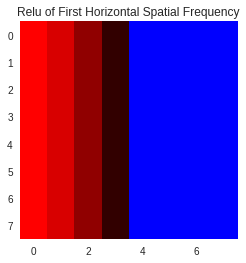Note that, as expected, the right side of the image, which would have originall been green (negative) is now blue (0). Note also the effect that this had on the DCT coefficients. Where the original image was generated by setting position $(0, 1)$ to 1, with all other coeffients set to 0, now much of the first tow has arbitrary values, including the DC coefficient. For another example, lets look at the first spatial frequency on the $y$-axis.

In :
dct_10 = np.zeros((8, 8))
dct_10[1, 0] = 1
im_10 = idct(dct_10)

relu_10 = relu(im_10)
show_image(relu_10)
plt.title('Relu of First Vertical Spatial Frequency')

relu_coeff_10 = dct(relu_10)
show_mat(relu_coeff_10)

 0.453 0 -0 0 0 0 -0 -0 0.5 0 -0 0 0 0 -0 -0 0.208 0 -0 0 0 0 -0 -0 -0 -0 0 -0 -0 -0 0 0 -0.037 -0 0 -0 -0 -0 0 0 0 0 -0 0 0 0 -0 -0 0.011 0 -0 0 0 0 -0 -0 0 0 -0 0 0 0 -0 -0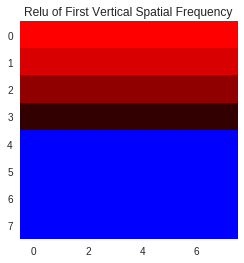As expected, this is simply the transpose of the previous example. What about something more complex? Let's try the coefficient at $(1, 1)$

In :
dct_11 = np.zeros((8, 8))
dct_11[1, 1] = 1
im_11 = idct(dct_11)

relu_11 = relu(im_11)
show_image(relu_11)
plt.title('Relu of (1, 1) DCT')

relu_coeff_11 = dct(relu_11)
show_mat(relu_coeff_11)

 0.411 0 0.188 0 -0.034 0 0.01 -0 0 0.5 0 -0 0 -0 0 0 0.188 0 0.086 0 -0.016 0 0.005 -0 0 -0 0 0 0 0 -0 0 -0.034 -0 -0.016 0 0.003 -0 -0.001 0 0 -0 0 0 -0 0 0 0 0.01 0 0.005 -0 -0.001 0 0 -0 -0 0 -0 0 0 0 -0 -0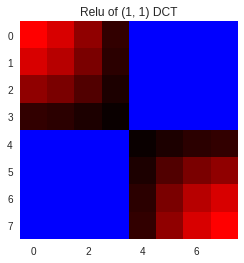It is clear that while the ReLu is a simple transformation, the DCT coefficients themselves are not changing in any simple way.

### Nonlinearity¶

Looking only at the equation for ReLu, we can see that it is piecewise linear. This is necessarily non-linear, and although its form is simple, it still fails to follow linearity rules in general. For example, consider the addition of the two ReLu images above. If ReLu were linear, then we would expect

$$r(a+b) = r(a) + r(b)$$
In :
relu_postadd = relu_01 + relu_10
relu_preadd = relu(im_01 + im_10)

plt.figure(figsize=(10, 10))

plt.subplot(1, 2, 1)
show_image(relu_postadd)
plt.title('r(a) + r(b)')

plt.subplot(1, 2, 2)
show_image(relu_preadd)
plt.title('r(a + b)');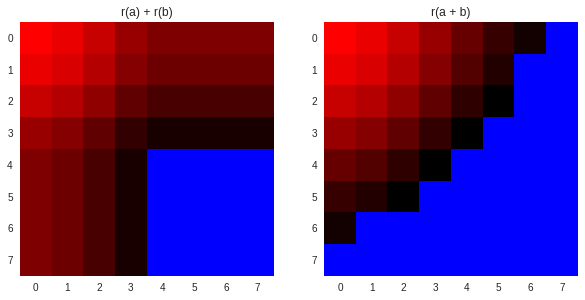Even in this simple case, the results are very different. Unfortunatly, because of this non-linearity, it is (most likely) not possible for obtain a simple closed form solution to find the ReLu by operating on DCT coefficients alone. Instead, we can use an approximation technique to get close to the true ReLu but with far fewer computations.

## Approximated Nonnegative Masking¶

Lets start by assuming we know the sign function of the original image, that is

$$\text{sgn}(x) = \left\{\begin{array}{lr} 1 & x > 0 \\ 0 & x = 0 \\ -1 & x < 0 \end{array}\right.$$

This gives all the information needed to compute the ReLu function of the original image, since it marks all negative values with a -1. Next lets consider a small change to this function

$$\text{nnm}(x) = \left\{\begin{array}{lr} 1 & x \geq 0 \\ 0 & x < 0 \end{array}\right.$$

Call this function the nonnegative mask of the image I, since it marks all negative values with a 0. At first glance this function may not seem useful since it is still non-linear. There is, however, a trick to computing an approximation of this function using the DCT coefficients, which is presented (in highly non-optimized code) below.

In :
def annm(dct_im, n_freqs):
sign_im = np.zeros_like(dct_im)
N = dct_im.shape
sign_lut = harmonics(N)
n = normalize(N)

sf = 0
for x in range(N):
for y in range(N):
accum = 0
for i in range(8):
for j in range(8):
if i + j <= n_freqs:
accum += n[i, j] * dct_im[i, j] * sign_lut[x, i] * sign_lut[y, j]

sign_im[x, y] = (1 / np.sqrt(2 * N)) * accum

mask = np.zeros_like(sign_im)
mask[sign_im >= 0] = 1
return mask


This algorithm builds a spatial domain approximation of the original image using the lowest n_freqs spatial frequencies only, then computes the non-negative mask. Since there are both horizontal and vertical spatial frequencies in a 2D signal, we need a single scalar measure of spatial frequency. The convention is to use the sum of $i$ and $j$ in the DCT equation. This is shown visually in the matrix below where each element is labeled with its spatial frequency.

In :
spatial_frequencies = np.zeros((8, 8))

for i in range(8):
for j in range(8):
spatial_frequencies[i, j] = i + j

show_mat(spatial_frequencies)

 0 1 2 3 4 5 6 7 1 2 3 4 5 6 7 8 2 3 4 5 6 7 8 9 3 4 5 6 7 8 9 10 4 5 6 7 8 9 10 11 5 6 7 8 9 10 11 12 6 7 8 9 10 11 12 13 7 8 9 10 11 12 13 14

For n_freqs=6, this would use 28 of the 64 DCT coefficients resulting $\frac{28}{64}$ or approximately 40% of the computations required to reconstruct the full image. There is a good theoretical reason to use this approximation: it is a well known result that given the DCT coefficients of an image, picking the lowest $m$ frequencies minimizes the least squares reconstruction error of the true image with respect to any other set of $m$ coefficients:

DCT Least Squares Approximation Theorem Consider the vector $x = [x_0, ..., x_n]$. Let $y = [y_0, ..., y_n]$ be the DCT coefficients of x. Then, for all integers $1 \leq m \leq n$, forming the vector p such that

$$p_m(t) = \frac{1}{\sqrt{n}}y_o + \sqrt{\frac{2}{n}}\sum_{k=1}^{m} y_k\cos\left(\frac{k(2t + 1)\pi}{2n}\right)$$

minimizes the least squares reconstruction error

$$e_m = \sum_{i=0}^{n} (p_m(i) - x_i)^2$$

The proof of this is simple and is a direct result of the DCT being an orthonormal transform and can be found in Chapter 6 of "A First Course in Applied Mathematics" by Jorge Rebaza (available here) among other places. Proof is given in the appendix to these notes.

In simpler words, what this theorem states is that given the only DCT coefficients of an image, the minimal error approximation using $m$ coefficients is formed by taking the $m$ lowest frequency coefficients as opposted to taking any other set of size $m$.

For a further characterization of the error of using the approximation, the true ReLu is computed on a randomized image and the result is compared to element-wise multiplication by the approximate nonnegative mask. A visual example is shown along with its error and then the process is carried out 1000 times for all spatial frequencies and the error is plotted. The example uses 6 spatial frequencies (again thats 28 matrix elements).

The images are generated as 4x4 random images and scaled to 8x8 using a nearest neighbor filter. This avoids bias from completely random images, which have very complex DCTs and generally do not represent the statistics of real images, while still allowing for a large random set of images. The error is computed as RMSE of the intesity value. Since the images are in the range $[-1, 1]$, this can be interpreted as $\text{intensity percentage} \times 2$. In other words, an image with RMSE=0.05 would mean pixel intensity differs by 2.5% on average. In reality, it is not that every pixel is off by some small percentage, but rather that certain pixels are wrong while most are correct.

In :
from scipy.misc import imresize

def error(a, b):
return np.sqrt(np.mean((a - b)**2))

im = np.random.rand(4, 4) * 2 - 1
im = imresize(im, (8, 8), interp='nearest', mode='F')
true_relu = relu(im)
dct_nnm = annm(dct(im), 6)

plt.figure(figsize=(20, 20))

plt.subplot(1, 3, 1)
show_image(im)
plt.title('Original Image')

plt.subplot(1, 3, 2)
show_image(true_relu)
plt.title('True ReLu')

plt.subplot(1, 3, 3)
show_image(im * dct_nnm)
plt.title('ReLu from ANNM')

print('Example Error: {}'.format(error(true_relu, im * dct_nnm)))

error_samples = np.zeros(15)
n_trials = 1
for i in range(15):
for j in range(n_trials):
im = np.random.rand(4, 4) * 2 - 1
im = imresize(im, (8, 8), interp='nearest', mode='F')
true_relu = relu(im)
dct_nnm = annm(dct(im), i)
error_samples[i] += error(im * dct_nnm, true_relu)

error_samples /= n_trials

plt.figure(figsize=(20, 5))
plt.plot(error_samples, c='b')
plt.title('Error of ANNM ReLu')
plt.ylabel('Error (Intensity)')
plt.xlabel('# Spatial Frequencies');


Example Error: 0.06129794863020826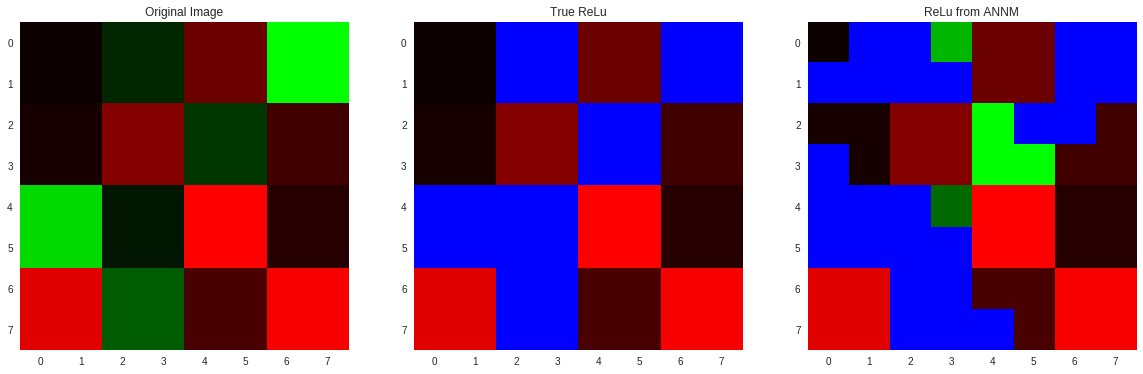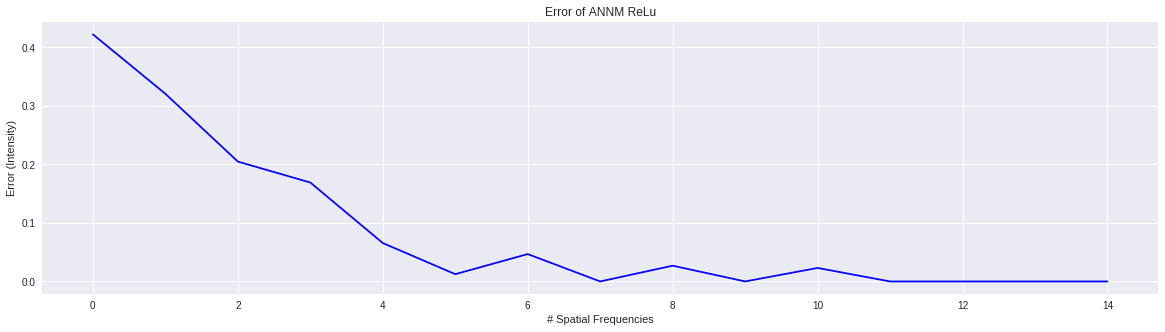As the graph above shows, using the lowest 6 DCT coefficients gives an RMSE of about 0.05, or 2.5% of intensity, while less than half of the computation time of fully reversing the DCT. The example shows the effect of this error where only 5 of the 64 pixels are incorrect. 4 of these errors leak a negative value with the remaining one masking a positive value.

## Approximating ReLu in the DCT Domain¶

There is still a big problem with what we have in the ANNM. While the ANNM masks spatial locations, we only have the DCT coefficents of the original image. The Half Spatial Masking algorithm is developed to allow this mask to be applied directly without undoing the DCT. This is a modification of the pixlewise product algorithm developed by Smith and Rowe (Pixelwise product algorithm from: Algorithms for Manipulating Compressed Images, Smith and Rowe 1993). While that algorithm allowed image pixels of two DCT transformed images to be multiplied, this allows for one spatial and one DCT image to be multiplied together.

Further optimization is used since masks contain only 0s and 1s. This takes the form of a sparsity check. If the mask location is zero, its contribution is not included. If it is 1, it is simply copied into the final sum. The result is a very fast algorithm even in the unoptiized form that it is presented below.

In :
def half_spatial_mask(x, y):
z = np.zeros_like(x)
N = x.shape

h = harmonics(N)

h[:, 0] *= 1 / np.sqrt(2)

for u1 in range(N):
for u2 in range(N):
for i in range(N):
for j in range(N):
if y[i, j] != 0:
for v1 in range(N):
for v2 in range(N):
z[u1, u2] += h[i, u1] * h[j, u2] * h[i, v1] * h[j, v2] * x[v1, v2]

return (1 / (2 * N)) * z


Next, the Half Spatial Masking algorithm is used to apply an ANNM. The result is compared to applying the ANNM directly in the spatial domain and to the true ReLu.

In :
im = np.random.rand(4, 4) * 2 - 1
im = imresize(im, (8, 8), interp='nearest', mode='F')

dct_im = dct(im)

true_nnm = np.zeros_like(im)
true_nnm[im > 0] = 1

im_relu = im * true_nnm

appx_nnm = annm(dct_im, 6)
appx_relu = half_spatial_mask(dct_im, appx_nnm)

spatial_appx = im * appx_nnm

plt.figure(figsize=(20, 20))

plt.subplot(1, 4, 1)
show_image(im)
plt.title('Original Image')

plt.subplot(1, 4, 2)
show_image(im_relu)
plt.title('True ReLu')

plt.subplot(1, 4, 3)
show_image(spatial_appx)
plt.title('Applying ANNM in the Spatial Domain')

plt.subplot(1, 4, 4)
show_image(idct(appx_relu).round(3))
plt.title('Applying ANNM using Half Spatial Masking');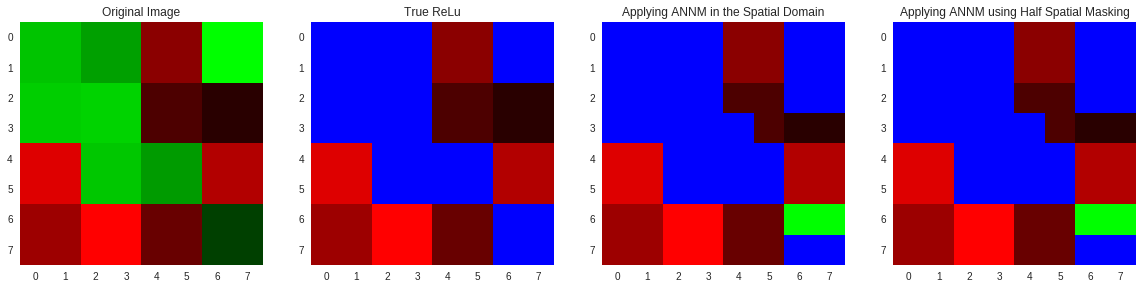## Approximated Spatial Masking¶

This algorithm is a case of Approximated Spatial Masking, which can be used to construct any spatial domain mask from DCT coefficients to within an approximation. Once the appropriate pixels are masked, linear transformations can be applied to them to compute any piecewise linear function of DCT coefficients. As far as I know it is the only attempt at computing a non-linear function on DCT coefficients.

One question that might be lingering after reading this is why use the mask at all? The naive algorithm would contruct the approximate spatial domain image in the same way, then simply apply the non-linear function to it and compute the DCT of the result. At first glance, this is actually even better because any arbitrary non-linear function could be computed, even ones not covered by masking.

The problem with this algorithm, however, comes from a subtle point of the DCT Least Squared Theorem. That is, given the points $x$, the DCT coefficients $y$ always interpolate the points $x$. When only the first $m$ coefficients are taken, they are no longer guarenteed to interpolate $x$, and often do not interpolate any of them, rather minimizing the error w.r.t. any other set of size $m$.

Let's examine this problem with the case of ReLu as it is the running example in these notes. By taking the non-negative mask of the approximate image, we only require the appximation to have gotten the sign of the true image correct. The values themselves then come from the original image, via the DCT coefficients, and are guarenteed to be correct. If we instead computed ReLu on the approximate image and used it directly, even positive pixels, e.g. pixels that were 1 on the mask and which should be untouched by the transformation, are probably not going to be correct. Put simpler: using the mask at least guarentees that even if the mask is incorrect, the actual values are still correct.

This is more than just a theoretical argument, it can be shown empirically. In the graph below, the error of the ReLu computed using ASM is shown along with the error of computing ReLu on the approximate image. Notice how the appoximate image always incurs a larger error for the same computational cost. The green line (the naive algorithm) has a larger error that decreases slower with increasing spatial frequencies when compared with the blue line (ASM method).

In :
def approx_relu(dct_im, n_freqs):
appx_im = np.zeros_like(dct_im)
N = dct_im.shape
sign_lut = harmonics(N)
n = normalize(N)

sf = 0
for x in range(N):
for y in range(N):
accum = 0
for i in range(8):
for j in range(8):
if i + j <= n_freqs:
accum += n[i, j] * dct_im[i, j] * sign_lut[x, i] * sign_lut[y, j]

appx_im[x, y] = (1 / np.sqrt(2 * N)) * accum

r = relu(appx_im)
return r

error_samples_anm = np.zeros(15)
error_samples_full = np.zeros(15)
n_trials = 1
for i in range(15):
for j in range(n_trials):
im = np.random.rand(4, 4) * 2 - 1
im = imresize(im, (8, 8), interp='nearest', mode='F')

true_relu = relu(im)

dct_nnm = annm(dct(im), i)
error_samples_anm[i] += error(im * dct_nnm, true_relu)

ar = approx_relu(dct(im), i)
error_samples_full[i] += error(ar, true_relu)

error_samples_anm /= n_trials
error_samples_full /= n_trials

im = np.random.rand(4, 4) * 2 - 1
im = imresize(im, (8, 8), interp='nearest', mode='F')
true_relu = relu(im)

dct_nnm = annm(dct(im), 6) * im
ar = approx_relu(dct(im), 6)

plt.figure()
show_image(im)
plt.axis('off')

plt.figure()
show_image(true_relu)
plt.axis('off')

plt.figure()
show_image(ar)
plt.axis('off')

plt.figure()
show_image(im * dct_nnm)
plt.axis('off')

plt.figure(figsize=(20,5))
plt.plot(error_samples_full, label='Approximated ReLu', c='g')
plt.plot(error_samples_anm, label='Approximated Spatial Mask ReLu', c='b')
plt.title('Approximating ReLu Directly vs ASM')
plt.xlabel('# Spatial Frequencies')
plt.ylabel('Error (Intensity)')
plt.legend();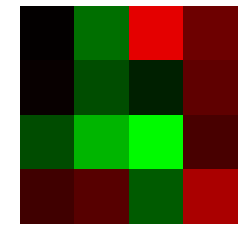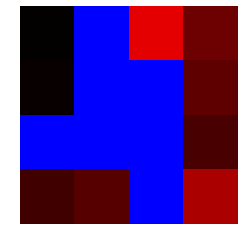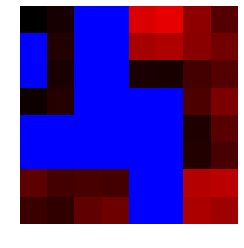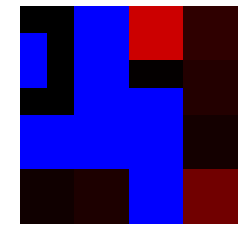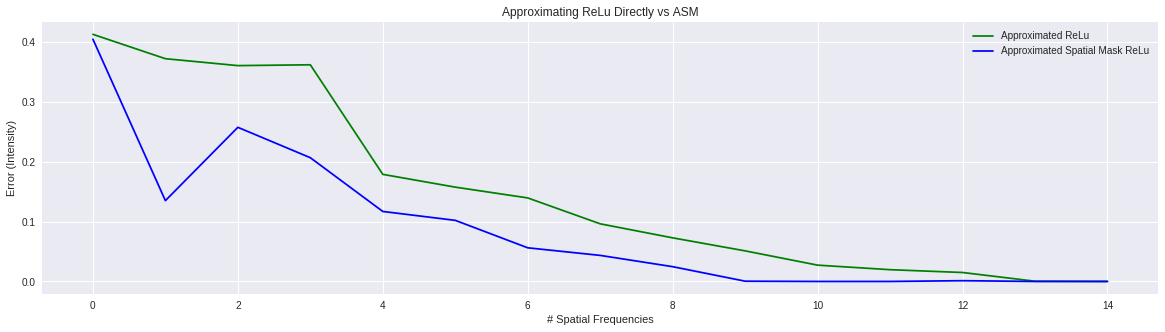## Multilinear Forms¶

In order to compute this algorithm efficiently on a GPU it must be vectorized. Here we show how to express the steps above as multilinear expressions that can be computed using the einsum functions. This follows from the notes on "Tensor Methods".

We start with the approximated nonnegative mask. The key point of this algorithm is computing an IDCT with the first $n$ spatial frequencies. This can be done easily by modifying the DCT tensor that was presented in the "Tensor Methods" notes. The new tensor simply does not set the coefficients for spatial frequencies higher than $n$, since they are initialized to zero. The following code implements a function which constructs this tensor given the desired number of spatial frequencies to use.

In :
def A(alpha):
if alpha == 0:
return 1.0 / np.sqrt(2)
else:
return 1

def D_a(n_freqs=14):
D_t = np.zeros([8, 8, 8, 8], dtype=np.float)

for i in range(8):
for j in range(8):
for alpha in range(8):
for beta in range(8):
if alpha + beta <= n_freqs:
scale_a = A(alpha)
scale_b = A(beta)

coeff_x = np.cos(((2 * i + 1) * alpha * np.pi) / 16)
coeff_y = np.cos(((2 * j + 1) * beta * np.pi) / 16)

D_t[i, j, alpha, beta] = 0.25 * scale_a * scale_b * coeff_x * coeff_y

return D_t


The mask is then computed from the resulting spatial approximation as usual. Note that in the original function, we were able to skip some steps of the loop if they should have zero coefficients. In the multilinear construction we cannot make such an optimization explicitly, but for sparse tensors it will happen automatically. Since dense tensors are used for the example, the multiplications by zero are actually carried out. The following code implements the vectorized (fast) ANNM algorithm and shows the result on an example image compared with the non-vectorized (slow) algorithm, they are identical.

In :
def multilinear_annm(dct_im, n_freqs):
D_n = D_a(n_freqs)
appx_im = np.einsum('ijab,ab->ij', D_n, dct_im)
mask = np.zeros_like(appx_im)
mask[appx_im >= 0] = 1
return mask

im = np.random.rand(4, 4) * 2 - 1
im = imresize(im, (8, 8), interp='nearest', mode='F')

dct_im = dct(im)

slow_annm = annm(dct_im, 6)
fast_annm = multilinear_annm(dct_im, 6)

plt.figure(figsize=(15, 15))

plt.subplot(1, 3, 1)
show_image(im)
plt.title('Original Image')

plt.subplot(1, 3, 2)
show_image(slow_annm)
plt.title('Slow ANNM')

plt.subplot(1, 3, 3)
show_image(fast_annm)
plt.title('Fast ANNM');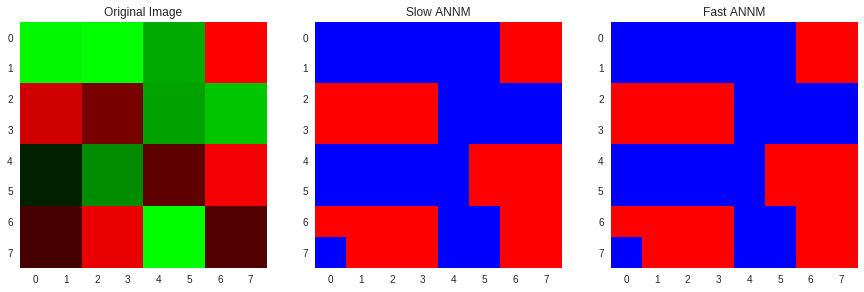Next, the half spatial masking algorithm must be implemented. This one is a little more complicated, but perhaps surprisingly, the solution is much cleaner since the original construction was multilinear. The naive algorithm would perform the following steps:

Given a DCT domain image $x$ and a spatial domain mask $y$

1. Take the IDCT of $x$ to give $x'$ in the spatial domain
2. Multiply the mask $y$ times $x'$ to mask the spatial domain pixels $z'$
3. Take the DCT of the result ($z'$) to give the final result $z$

This is actually exactly what the half spatial masking algorithm is doing, however since all these operations are linear the coefficients can be multiplied out beforehand and reduced to a simpler expression. These steps also have multilinear constructions, but there is one catch, which is the elementwise multiply. Consider the following multilinear expressions for the above steps:

1. $D^{a,b}_{i, j}x_{a, b} = x'_{i, j}$
2. $x'_{i, j} y_{i, j} = z'_{i, j}$
3. $D^{i, j}_{u, v} z'_{i, j} = z_{u, v}$

Note the middle step, in previous examples, any repeated indices were summed out. In this case, even though the indices are repeated on the left, they are explicitly preserved on the right, indicating an elementwise multiplication. The final multilinear expression is

$$D^{a,b}_{i,j}x_{a,b}y_{i,j}D^{i,j}_{u,v} = z_{u,v}$$

However, we can make one final optimization by commuting the DCT tensor $D$ as follows:

$$D^{a,b}_{i,j}x_{a,b}y_{i,j}D^{i,j}_{u,v} = z_{u,v} = \\ D^{a,b}_{i,j}D^{i,j}_{u,v}x_{a,b}y_{i,j} = \\ \left[D^{a,b}_{i,j}D^{i,j}_{u,v}\right]x_{a,b}y_{i,j} = \\ H^{i, j, a, b}_{u, v}x_{a,b}y_{i,j} = z_{u,v}$$

The tensor $H$, called the Harmonic Mixing Tensor, permutes the products of the spatial frequency harmonics for us, and can be precomputed to give a highly efficient form for the half spatial masking algorithm. It is again worth noting that in the original loop-based half spatial masking algorithm, multiplying by the mask was avoided entirely since the mask value is either 0, and the pixel can be ignored, or 1 and the pixel can be included with no extra multiply. In the dense version, these extra multiplies can't be ignored.

The following code implements the above eqution and the result is shown along with the original slow version.

In :
D_14 = D_a(14)
Hm = np.einsum('ijab,ijuv->ijabuv', D_14, D_14)

def multilinear_hsm(x, y):
return np.einsum('ijabuv,ab,ij->uv', Hm, x, y)

slow_relu = half_spatial_mask(dct_im, fast_annm)
fast_relu = multilinear_hsm(dct_im, fast_annm)

plt.figure(figsize=(20, 20))

plt.subplot(1, 4, 1)
show_image(im)
plt.title('Original Image')

plt.subplot(1, 4, 2)
show_image(fast_annm)
plt.title('ANNM')

plt.subplot(1, 4, 3)
show_image(idct(slow_relu).round(3))
plt.title('Slow Relu')

plt.subplot(1, 4, 4)
show_image(idct(fast_relu).round(3))
plt.title('Fast Relu');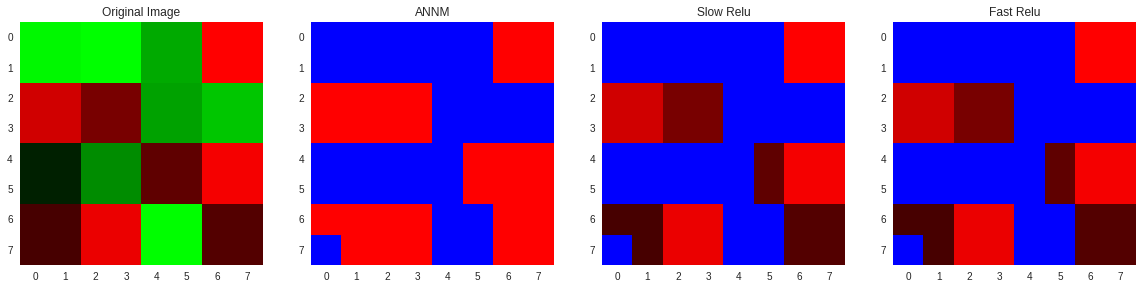© 2018 Max Ehrlich